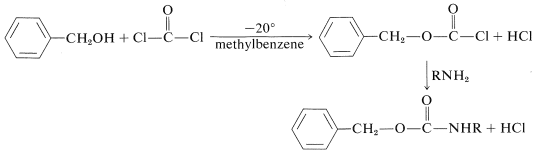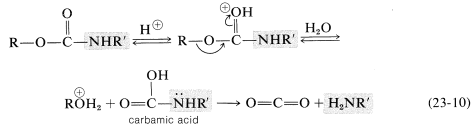# 23.13: Protection of Amino Groups in Synthesis

$$\newcommand{\vecs}{\overset { \rightharpoonup} {\mathbf{#1}} }$$ $$\newcommand{\vecd}{\overset{-\!-\!\rightharpoonup}{\vphantom{a}\smash {#1}}}$$$$\newcommand{\id}{\mathrm{id}}$$ $$\newcommand{\Span}{\mathrm{span}}$$ $$\newcommand{\kernel}{\mathrm{null}\,}$$ $$\newcommand{\range}{\mathrm{range}\,}$$ $$\newcommand{\RealPart}{\mathrm{Re}}$$ $$\newcommand{\ImaginaryPart}{\mathrm{Im}}$$ $$\newcommand{\Argument}{\mathrm{Arg}}$$ $$\newcommand{\norm}{\| #1 \|}$$ $$\newcommand{\inner}{\langle #1, #2 \rangle}$$ $$\newcommand{\Span}{\mathrm{span}}$$ $$\newcommand{\id}{\mathrm{id}}$$ $$\newcommand{\Span}{\mathrm{span}}$$ $$\newcommand{\kernel}{\mathrm{null}\,}$$ $$\newcommand{\range}{\mathrm{range}\,}$$ $$\newcommand{\RealPart}{\mathrm{Re}}$$ $$\newcommand{\ImaginaryPart}{\mathrm{Im}}$$ $$\newcommand{\Argument}{\mathrm{Arg}}$$ $$\newcommand{\norm}{\| #1 \|}$$ $$\newcommand{\inner}{\langle #1, #2 \rangle}$$ $$\newcommand{\Span}{\mathrm{span}}$$$$\newcommand{\AA}{\unicode[.8,0]{x212B}}$$

We have mentioned previously that it may be difficult to ensure selective chemical reaction at one functional group when other functional groups are present in the same molecule. Amino groups are particularly susceptible to reactions with a wide variety of reagents, especially oxidizing reagents, alkylating reagents, and many carbonyl compounds. Therefore, if we wish to prevent the amino group from undergoing undesired reaction while chemical change occurs elsewhere in the molecule, it must be suitably protected. There is more documented chemistry on methods of protecting amino groups than of any other functional group. This is because peptide synthesis has become very important and, as we shall see in Chapter 25, it is not possible to build a peptide of specific structure from its component amino acids unless the amino groups can be suitably protected. Therefore we now will consider the more useful protecting groups that are available - how they are introduced and how they are removed.

## Protonation

It should be clear that the reactivity of amines normally involves some process in which a bond is made to the unshared electron pair on nitrogen. Therefore any reaction of an amine that reduces the reactivity of this electron pair should reduce the reactivity of the nitrogen atom. The simplest way to do this would be to convert the amine to an ammonium salt with an acid. Protonation amounts to protection of the amine function:

$\ce{RNH_2} + \ce{HX} \rightleftharpoons \ce{R} \overset{\oplus}{\ce{N}} \ce{H_3} \: \overset{\ominus}{\ce{X}}$

Examples are known in which amines indeed can be protected in this manner, but unless the acid concentration is very high, there will be a significant proportion of unprotected free base present. Also, many desirable reactions are not feasible in acid solution.

## Alkylation

A related protection procedure is alkylation (Equations 23-8 and 23-9), which is suitable for primary and secondary amines:

$\ce{R'NH_2} + \ce{RX} \rightarrow \ce{R'NHR} + \ce{HX} \tag{23-8}$

$\ce{R'_2NH} + \ce{RX} \rightarrow \ce{R'_2NR} + \ce{HX} \tag{23-9}$

At first glance, you may not consider that such reactions achieve protection because there is an electron pair on nitrogen in the products. However, it a suitably bulky alkylating agent, $$\ce{RX}$$, is used the reactivity of the resulting alkylated amine can be reduced considerably by a steric effect. The most useful group of this type is the triphenylmethyl group $$\ce{(C_6H_5)_3C}-$$, which can be introduced on the amine nitrogen by the reaction of triphenylmethyl chloride ("trityl" chloride) with the amine in the presence of a suitable base to remove the $$\ce{HCl}$$ that is formed:The triphenylmethyl group can be removed from the amine nitrogen under very mild conditions, either by catalytic hydrogenation or by hydrolysis in the presence of a weak acid:## Acylation

One useful way of reducing the basicity and nucleophilicity of an amine nitrogen is to convert it to an amide by treatment with an acid chloride or acid anhydride (Section 18-7):

\begin{align} \ce{RNH_2} + \ce{CH_3COCl} &\rightarrow \ce{RNHCOCH_3} + \ce{HCl} \\ \ce{RNH_2} + \ce{(CH_3CO)_2O} &\rightarrow \ce{RNHCOCH_3} + \ce{CH_3CO_2H} \end{align}

The reduced reactivity is associated with the stabilization produced by the attached carbonyl group because of its ability to accept electrons from the nitrogen atom. This can be seen clearly in valence-bond structures $$9a$$ and $$9b$$, which show electron delocalization of the unshared pair of the amide function:The stabilization energy (SE) of a simple amide grouping is about $$18 \: \text{kcal mol}^{-1}$$, and if a reaction occurs in which the amide nitrogen acts as an electron-pair donor, almost all of the electron delocalization of the amide group is lost in the transition state:This loss in stabilization energy at the transition state makes an amide far less nucleophilic than an amine.

The most common acylating agents are the acyl chlorides and acid anhydrides of ethanoic acid and benzoic acid. The amine can be recovered from the amide by acid- or base-catalyzed hydrolysis:Another useful protecting group for amines has the structure $$\ce{R-O-CO}-$$. It differs from the common acyl groups of the type $$\ce{R-CO}-$$ in that it has the alkoxycarbonyl structure rather than an alkylcarbonyl structure. The most used examples are:The phenylmethoxycarbonyl (benzyloxycarbonyl) group can be introduced by way of the corresponding acyl chloride, which is prepared from phenylmethanol (benzyl alcohol) and carbonyl dichloride:The tert-butoxycarbonyl group cannot be introduced by way of the corresponding acyl chloride because $$\ce{(CH_3)_3COCOCl}$$ is unstable. One of several alternative derivatives is the azide, $$\ce{ROCON_3}$$:Although these protecting groups may seem bizarre, their value lies in the fact that they can be removed easily by acid-catalyzed hydrolysis under very mild conditions. The sequence of steps is shown in Equation 23-10 and involves proton transfer to the carbonyl oxygen and cleavage of the carbon-oxygen bond by an $$S_\text{N}1$$ process ($$\ce{R} =$$ tert-butyl) or $$S_\text{N}2$$ process ($$\ce{R} =$$ phenylmethyl). The product of this step is a carbamic acid. Acids of this type are unstable and readily eliminate carbon dioxide, leaving only the free amine (also see Section 23-12E):The benzyloxycarbonyl group, but not the tert-butoxycarbonyl group, may be removed by catalytic hydrogenation. Again a carbamic acid is formed, which readily loses $$\ce{CO_2}$$:## Sulfonylation

A sulfonyl group, $$\ce{RSO_2}-$$, like an acyl group, $$\ce{R-CO}-$$ or $$\ce{RO-CO}-$$, will deactivate an attached nitrogen. Therefore amines can be protected by transformation to sulfonamides with sulfonyl chlorides (Section 23-9C):

$\ce{C_6H_5SO_2Cl} + \ce{RNH_2} \rightarrow \ce{C_6H_5SO_2NHR} + \ce{HCl}$

However, sulfonamides are much more difficult to hydrolyze back to the amine than are carboxamides. In peptide synthesis (Section 25-7C) the commonly used sulfonyl protecting groups are 4-methylbenzenesulfonyl or 4-bromobenzenesulfonyl groups. These groups can be removed as necessary from the sulfonamide by reduction with sodium metal in liquid ammonia:$$^6$$This abbreviation is approved by the IUPAC-UIB Commission on Biochemical Nomenclature and is typical of the kind of "alphabet soup" that is making biochemistry almost completely unintelligible without a glossary of approved (and unapproved) abbreviations at hand at all times. We shall make minimum use of such designations. You will remember we already use $$\ce{Z}$$ for something else (Section 19-7).